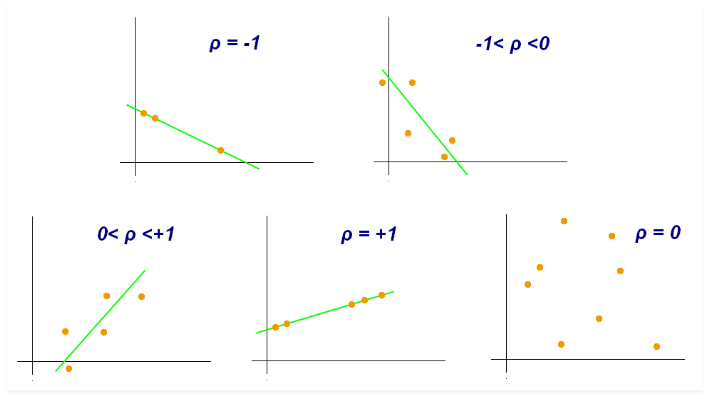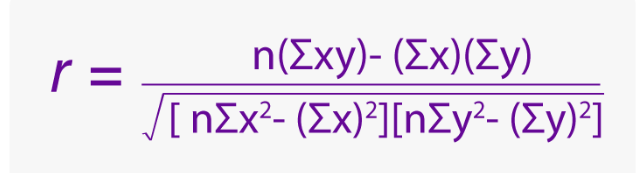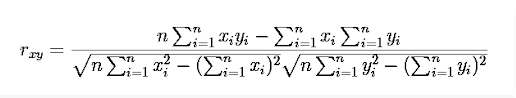Jet Set Go! All about Aeroplanes Jet Set Go! All about Aeroplanes

# Correlation Coefficient

In Statistics, the correlation coefficient is used to measure the extent of the relationship between two variables. It is scaled between the range, -1 and +1. The coefficient of correlation between two intervals or ratio level variables is represented by ‘r’. When the value of r is near to zero (0), then it is considered that there is little relation between the variables. The more the value of r far from zero, both at the negative and positive direction, the greater is the relationship between the variables. If the value of r is zero, then there is no relation between the variables.

The examples of the correlation coefficient can be seen widely in the finance sectors. For example, as the oil prices increase the oil-producing companies profit more, which determines the correlation between the two quantities.

## Correlation Coefficient Definition

As stated earlier, the extent of the relationship between any two variables is defined by the correlation coefficient. It is also called the cross-correlation coefficient, Pearson correlation coefficient (PCC), Pearson’s r, the Pearson product-moment correlation coefficient (PPMCC), or the bivariate correlation.

Below is the scatter plot explaining the correlation between variables in terms of the coefficient.There are many types of correlation coefficient. One of the commonly used is Pearson’s correlations (also called Pearson’s R) in linear regression. When we speak about the correlation coefficient in statistics, it is Pearson Coefficient only.

### Correlation Coefficient Formula

Let us discuss here the correlation coefficient formula. Suppose x and y are the two variables which are related to each other. The extent to find the relationship between the two variables is given by the Pearson Coefficient ‘r’. And the formula to find the extent is equal to:This is the formula for Pearson’s correlation coefficient, where;

n = Data quantity or number of data available

Σx = Total of the First Variable Value

Σy = Total of the Second Variable Value

Σxy = Sum of the Product of First & Second Value

Σx2 = Sum of the Squares of the First Value

Σy2 = Sum of the Squares of the Second Value

But apart from this, we also have a linear coefficient correlation formula, which is given by;Where n is the number of observations and xi and yi are the variables.

Also, we can calculate the value of the sample and population correlation coefficients by using the formulas given below;

For Sample Correlation:

rxy = Sxy /SxSy

Where Sx and Sy are the sample standard deviations and Sxy is the sample covariance.

For Population Correlation:

ρxy = σxyxσy

σx and σy are the population standard deviations

σxy is the population covariance

This is also known as the correlation coefficient equation.

### Correlation Coefficient Meaning and Examples

The exact meaning of the correlation coefficient is explained here on the basis of the value of r varying between -1 and +1. Let us discuss here:

• If r =1, that means for each positive increase in one variable, there is a positive increase in another variable too, in a fixed proportion. For example, the size of the cloth increases in correlation with the height of the person.
• If r = -1, that means for each positive increase in one variable, there is a negative decrease in another variable too, in a fixed proportion. For example, the distance decreases in correlation with the increasing speed vehicle.
• If r = 0, there is no increase or decrease of another variable with respect to first. They are not related to each other here.